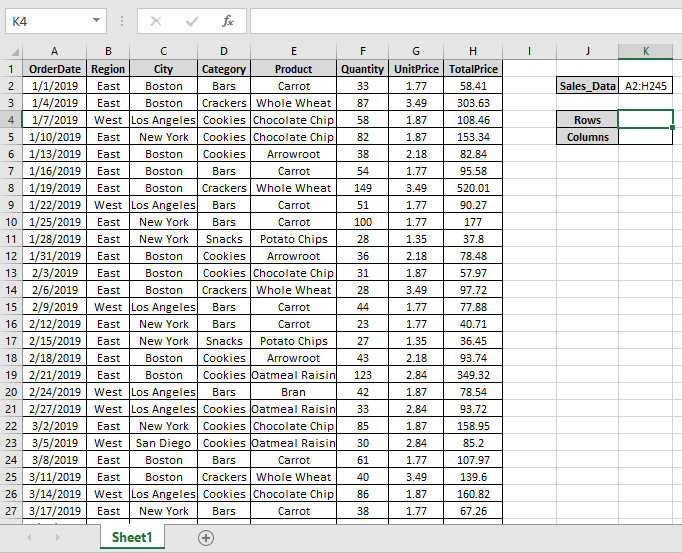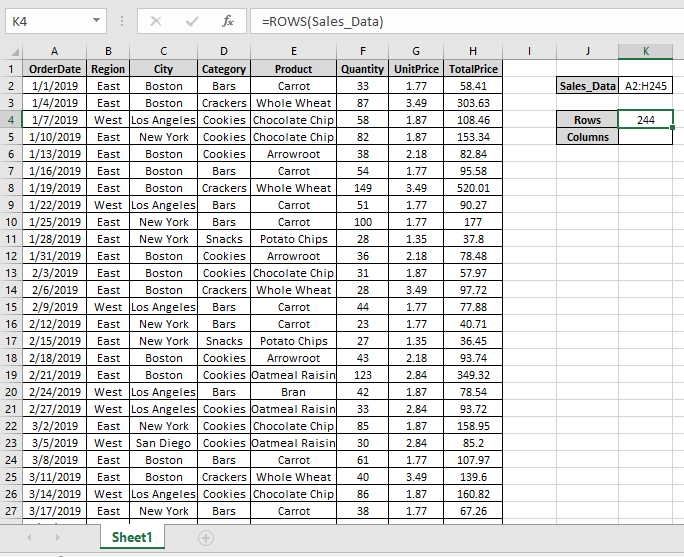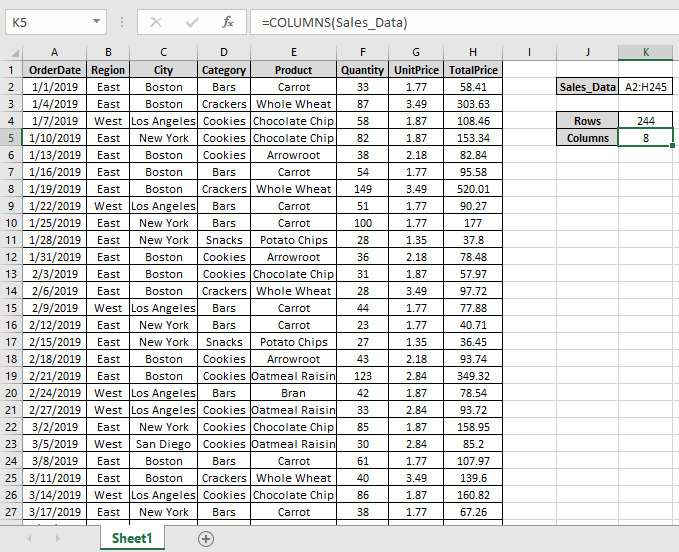How to Count table rows & columns in Excel

In simple words, while working with large data in Excel we need to find the number of rows or columns in excel table.

The ROWS function in excel returns the number of rows in an array.
Syntax:

=ROWS(array)

The COLUMNS function in excel returns the number of columns in an array.
Syntax:

=COLUMNS(array)

Let’s understand this function using it in an example.

Here we have large data A2:H245 named Sales_DataHere we need to find out the number of rows & columns of Sales_Data table in Excel.

Use the formula to get the number of rows

=ROWS(A2:H245)

A2:H245 : Sales_Data table as an array.We got the number of rows of the Sales_Data.
Use the formula to get the number of rows

=COLUMNS(A2:H245)

A2:H245 : Sales_Data table as an array.As you can see the ROWS & COLUMNS functions returns the number of rows & columns of table.

Hope you understood how to use ROWS function and COLUMNS function in Excel. Explore more articles on Excel cell info function functions here. Please feel free to state your query or feedback for the above article.

Related Articles :

How to use the CELL function in Excel

How to use the ROW function in Excel

How to use the COLUMN Function in Excel

Popular Articles :

50 Excel Shortcut to Increase Your Productivity : Get faster at your task. These 50 shortcuts will make you work even faster on Excel.

How to use the VLOOKUP Function in Excel : This is one of the most used and popular functions of excel that is used to lookup value from different ranges and sheets.

How to use the COUNTIF function in Excel : Count values with conditions using this amazing function. You don't need to filter your data to count specific values. Countif function is essential to prepare your dashboard.

How to use the SUMIF Function in Excel : This is another dashboard essential function. This helps you sum up values on specific conditions.

Terms and Conditions of use

The applications/code on this site are distributed as is and without warranties or liability. In no event shall the owner of the copyrights, or the authors of the applications/code be liable for any loss of profit, any problems or any damage resulting from the use or evaluation of the applications/code.